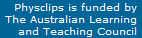## Molecules and photons

This page supports the multimedia tutorial The Nature of Light.

### Quantisation on the molecular scale: e.g. water

When we drink a glass of water, it seems to be a continuum: it seems as though we could divide it into ever smaller quantities. Yet, at the molecular scale, we know it is quantised: it comes in discrete units called molecules. It's worth being quantitative about this, which is the purpose of this illustration.

We begin with 18 g, which is one gram-mole or 6x1023 molecules. Dividing it in half gives 9 g, dividing in half again gives 4.5 g, and so on. Ten divisions (210 is about a thousand) takes us to 18 μl, and we can show a few further halvings with the microsyringe. But to get to molecular quantities, we need very many more divisions.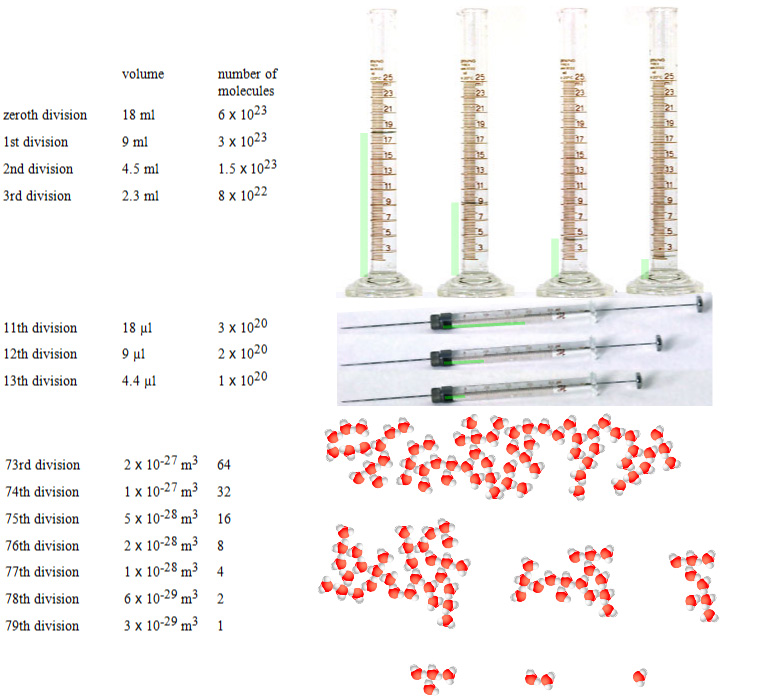How many times must we divide a macroscopic volume of water before we notice that it is not continuous, but molecular? Here we start wtih 18 ml, which is one mole and make successive divisions by 2.

270 is about 6 X 1023 so, after 79 divisions by two, we have reduced a mole of water to a single molecule. It is not surprising that we don't notice the discrete nature of water at the macroscopic level.

(Today, scanning tunnelling microscopes show images of individual atoms and everyone knows of them – which is why I began with quantisation of matter before talking of the quantisation of light. Further, the existence of atoms was postulated by Leucippus and Democritus in the 5th century BC. But persuasive evidence for atoms and molecules is not so ancient. Here is a brief history: Robert Boyle, in the 17th century, had evidence from his studies of gases, elements and compounds. Lavoisier, a century later, listed 28 elements. In the early 19th century Dalton and Proust developed atomic theory and determined relative molecular masses. Robert Brown in 1827 was perhaps the first to see molecular effects, deducing the motion of tiny pollen grains were due to collisions with molecules. Later that century, Mendelev wrote the periodic table. In 1912, Millikan showed that electric charge is quantised and Aston soon developed the mass spectrograph to measure the charge mass ratio of ions.)

A molecule is the smallest possible quantity of water – any further division gives oxygen and hydrogen, or a collection of subatomic particles. Is there a smallest possible amount of light?

### Light and photons

What happens at the molecular level when light interacts with materials? How does light interact with an electron in a solar panel, giving it enough energy to cross a diode? How does light interact with a molecule of photorhodopsin to put it into its excited state and thus to contribute to vision?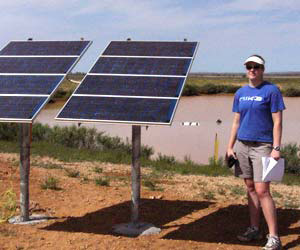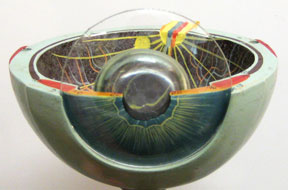Light interacts with electrons in a photoelectric panel and with photorhodopsin molecules in the retina of the eye.

Each electron is excited by its interaction with one photon, and that the photon is an indivisible quantity of light. We'll discuss this in more detail in a later chapter, but let's continue with an overview.

### Photon energy depends on wavelength

Light comes in photons, and each photon has an indivisible quantity (quantum) of energy. We'll see in a later chapter that the energy of a photon is
E  =  hf  =  hc/λ
where h is Planck's constant, f is the frequency and λ is the wavelength. Here is a plot of E(λ) that includes UV, visible and IR ranges.Energy per photon E  =  hc/λ

Here we see that, for visible light, photons have energies of a few electron volts each. (One photon has enough energy to excite an electron over a diode reverse biased by a few volts.) There is more discussion and many more examples in The electromagnetic spectrum.

### The photoelectric effect

So, what is the direct evidence for photons? Here is our version of a famous experiment. A negative electric charge is placed on the aluminium bar by rubbing a plastic rod on cloth, then touching rod to bar. The bar is mounted on an electroscope, which indicates the electrical potential of the bar: the ends of the rotating arm are repelled from their vertical support, so the inclination of the arm indicates its potential.

Under the visible light in the lab, any discharge of electrons from the bar is sufficiently slow that we do not notice the electrical potential changing.

 The photoelectric effect: an aluminium bar mounted on an electroscope is exposed first to visible light, then to ultraviolet light.

Exposure to ultraviolet light causes immediate discharge: the energy required to release an electron from Aluminium is about 4 eV. As the graph above shows, visible photons do not have sufficient energy to eject an electron, so the bar remains charge. Even photons in the near UV have sufficient energy, and so the bar is quickly discharged.

The photoelectric effect refers to the emission of electrons from the surface of a conductor (usually a metal) by incoming electromagnetic radiation.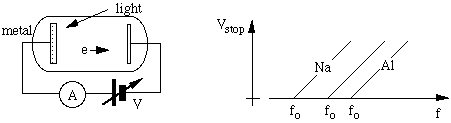A clean metal surface, often in vacuum, is exposed to light whose wavelength or frequency may be controlled. A nearby electrode can receive the emitted electrons and so allow a current, which may be measured. A variable potential difference may be applied to stop the current. For a given metal, there is a minimum frequency fo (ie a maximum wavelength) of light that will cause electrons to be emitted. For light (or UV radiation) with higher frequency, the stopping voltage increases linearly with frequency f, as sketched. More reactive metals have lower fo.

This phenomenon was investigated experimentally by Philipp Lenard and then later and more precisely by Robert Millikan. Both received Nobel prizes for the work. The photoelectric effect was explained by Albert Einstein in work for which he received the Nobel prize in 1921.

#### The quantisation of emitted radiation and black body radiation

Black body radiation is (by definition) radiation in thermal equilibrium with its container. So let us think of a box with hot walls giving off and receiving photons at an equal rate. If we open a small window in the box, the radiation coming out will have the same spectrum, and this is what we measure with our spectrometer to determine the black body radiation spectrum. The spectral radiancy is given by Planck's radiation law, which was initially empirical.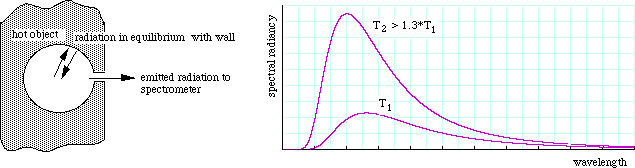On the left is a sketch of the experimental apparatus used to observe black body radiation. An object of controlled temperature T contains a cavity, joined to the outside by a small hole. If the hole is very small, the radiation in the cavity comes to equilibrium with the walls. The hole allows a small fraction of the radiation to pass to a spectrometer. On the right is a plot of Planck's radiation law for two temperatures. Note that the wavelength for maximum emission becomes shorter (higher frequency) for higher temperature. Note also the strong dependence on temperature of the total emission. The radiancy is the power emitted per unit area per increment of wavelength and so has units of W.m-3.

Note that the peak of the curve moves to the left as the temperature increases: hotter objects output a larger fraction of their electromagnetic radiation at shorter wavelengths. This displacement of the peak of the curve is called Wien's displacement law. After taking the derivative of Planck's radiation law and setting it to zero, one finds an expression for the wavelength λmax at which the radiation is a maximum. It is related to the temperature T of the black body by the simple equation

λmax = (2.9 x 10-3 mK)/T.
What we want to know is the distribution of energy among the photons in the box. To do this properly would require a bit of quantum mechanics and statistical mechanics. But your question is simpler: why does the distribution go from zero at very low frequency to a peak at some frequency and then back to zero at high frequency?

At low frequencies, the wavelengths are long. There are relatively few ways in which standing waves can fit into the box if their wavelengths are long. So, if the energy is shared among the different possible standing waves, we should expect the radiation intensity to go to zero at low frequency and to increase with increasing frequency. This is the classical result.

But now let's add the quantum hypothesis, that radiation energy is quantised and comes in photons that have an energy hf. In thermal equilibrium, the atoms and electrons of the wall have thermal motion. At sufficiently high frequency, few atoms or electrons will have enough energy to emit a photon with energy hf. Note how this depends on the quantisation hypothesis: if you could have radiation with frequency f and an energy less than hf, then the walls would emit high frequencies like crazy. They don't because they have to emit only whole photons.

So, at low frequency, the long wavelength limits the number of possible photons. At high frequency, the high energy makes emission unlikely. So the distribution goes to zero at both f = 0 and f = infinity, and has a maximum in between.

Incidentally, the frequency for maximum energy is proportional to the temperature of the walls. Wien's Law. The typical energy of any motion is kT/2, where k is Boltzmann's constant and T is the temperature. (k = R/NA where R is the gas constant and NA is Avagadro's number.)

There is more discussion and many more examples in The electromagnetic spectrum. The following link takes you back to the multimedia tutorial The Nature of Light.This work is licensed under a Creative Commons License.